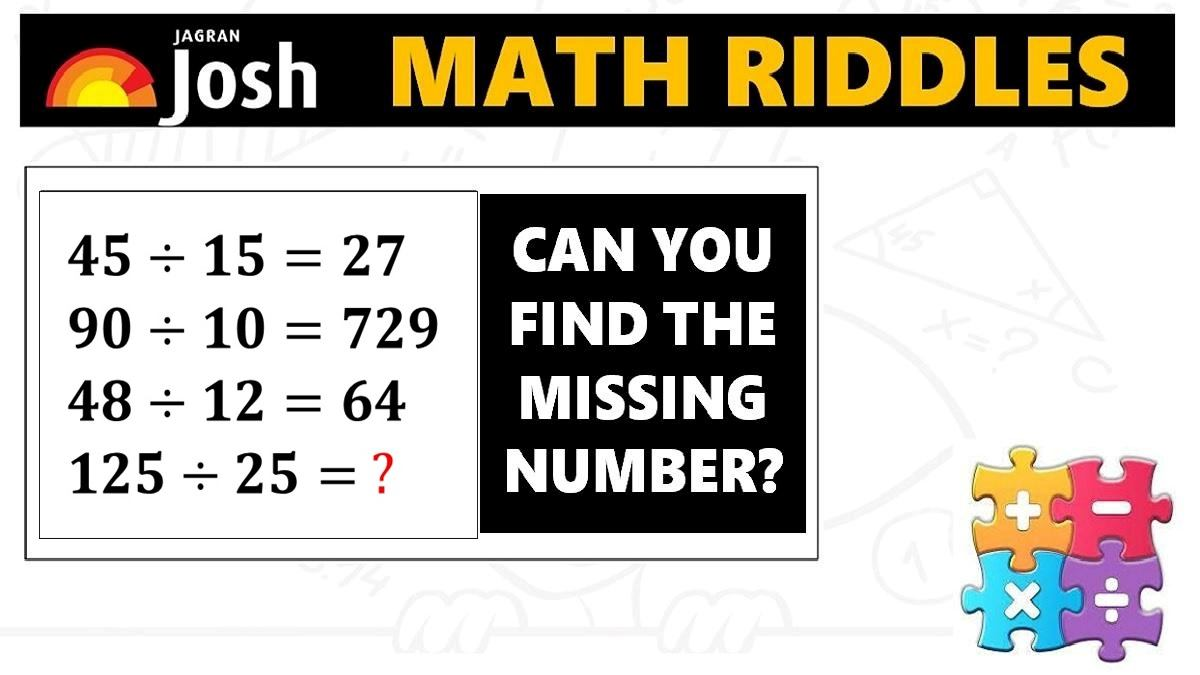# Math Riddles: Only 1% genius can solve these mathematics puzzles

Math Riddles Challenge: Only a 100% genius can solve these mathematics puzzle in less than 1 minute each?Math Riddles: Only 1% genius can solve these mathematics puzzles

Math Riddles: Only 1% genius have been able to solve these tricky mathematics puzzles. Do you have the IQ to find the right answer of each mathematics puzzle below in less than 1 minute each? If you are looking for preparation booster for the quantitative aptitude and reasoning ability sections in competitive government civil services exams, defence and banking exams, give these puzzles a try.

Explanation:

Explanation:

## Tell us in comments: Did you solve these mathematics puzzles in less than 1 minute each?

Check out more math puzzles!

Also Read: Math Riddles: Check Your Brain Power, Solve these Mathematics Puzzles

Also Read: Math Riddles: Can You Find The Next Number In These Math Puzzle Series?

Also Read: Math Riddles: Only 1 out 5 People Can Solve These 5-Minute Maths Puzzles

Get the latest General Knowledge and Current Affairs from all over India and world for all competitive exams.
खेलें हर किस्म के रोमांच से भरपूर गेम्स सिर्फ़ जागरण प्ले पर